# lift

(redirected from Lift (disambiguation))
Also found in: Dictionary, Thesaurus, Medical, Legal, Financial.

## lift

1.
a. Brit a platform, compartment, or cage raised or lowered in a vertical shaft to transport persons or goods in a building
2. the force required to lift an object
3.
a. the component of the aerodynamic forces acting on a wing, etc., at right angles to the airflow
b. the upward force exerted by the gas in a balloon, airship, etc.
4. See airlift
Collins Discovery Encyclopedia, 1st edition © HarperCollins Publishers 2005
The following article is from The Great Soviet Encyclopedia (1979). It might be outdated or ideologically biased.

## Lift

For a body moving in a liquid or gaseous medium, the lift acting on the body is the component of the medium’s total pressure force on the body in the direction perpendicular to the velocity of the body—or the velocity of the body’s center of gravity if the body is moving nontranslationally. The lift results from the unsymmetrical flow of the medium around the body. For example, in the case of flow around an airplane wing (Figure 1), the particles of the medium that flow past the lower surface traverse in the same period of time a shorter path than do the particles flowing past the upper, more convex surface. The velocity along the lower surface is consequently less than the velocity along the upper surface. According to Bernoulli’s equation, however, the pressure of the medium is greater where the velocity of the particles is smaller. The pressure of the medium is thus greater on the lower surface of the wing than on the upper surface, and lift results.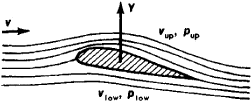< vup,="" and="" the="" pressure="" plow=""> pup; (y) lift on the wing"" height="102" width="252"/>
Figure 1. Flow around an airplane-wing profile: the velocity vlow < vup, and the pressure plow > pup; (y) lift on the wing

The unsymmetrical flow around the wing can be viewed as the result of superimposing on a symmetrical stream a circulatory flow around the wing contour. Along the more convex surface the flow moves in the direction of the mainstream, and an increased velocity results. Along the less convex surface the circulatory flow is directed against the mainstream, with a resulting decreased velocity. The lift Y will then depend on the value of the velocity circulation Γ. According to Zhukovskii’s theorem, when an ideal incompressible fluid moves in a two-dimensional parallel flow around the portion of a wing of length L, Y = ρvΓ L, where ρ is the density of the medium and v is the velocity of the oncoming flow.

Since Γ has the dimension of [vl], the lift can be expressed by the equation Y= cy ρSv2/2, which is commonly used in aerodynamics. Here S is a representative area of the body—for example, the plan area of the wing; cy is the nondimensional lift coefficient, which depends on the shape of the body, the body’s orientation in the medium, the Reynolds number Re, and the Mach number M. The value of cy can be determined by theoretical calculation or experimental means. Thus, according to Zhukovskii’s theory, for a wing in a two-dimensional parallel flow cy = 2m(α — α0), where α is the angle of attack (the angle between the direction of the velocity of the oncoming flow and the wing chord), α0 is the zero-lift angle, and m is a factor dependent only on the shape of the wing profile—for example, for a thin cambered plate, m = π. In the case of a wing of finite span l, m = π/(1 — 2/λ), where λ = l2/S is the wing’s aspect ratio.

Owing to viscosity, in a real fluid the value of m is smaller than the theoretical value, and the difference increases as the thickness ratio is increased. The value of α0 is also less than the theoretical value. Moreover, as α increases, the dependence of cy on α (Figure 2) ceases to be linear, and the quantity dcy/dα decreases monotonically, becoming zero at the angle of attack αcr corresponding to the maximum value cymax of the lift coefficient. A further increase in α causes a decrease in cy owing to separation of the boundary layer from the upper surface of the wing. The quantity cymax is of great importance, since the larger it is, the lower the plane’s takeoff and landing speeds.

At high but subcritical speeds—that is, at speeds for which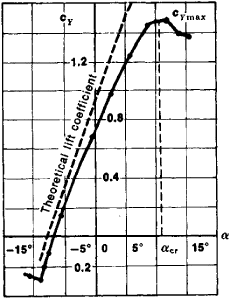Figure 2. Dependence of cy on α

M < Mcr where Mcr is the value of the Mach number of the oncoming flow at which the local values of the Mach number near the surface of the profile are equal to unity—the compressibility of the gas becomes significant. In the case of slightly cambered and thin profiles, the compressibility for small angles of attack may be taken into account by assuming, as an approximation, that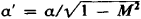and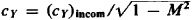.

At supersonic speeds the nature of the flow around a body is considerably different. Thus, in the case of flow around a flat plate, rarefaction waves form at the leading edge on the upper surface, and a shock wave forms on the lower surface (Figure 3).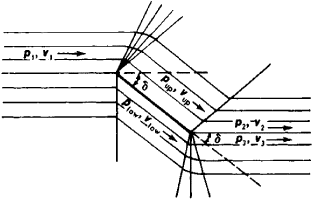Figure 3. Schematic of supersonic flow around a plate: vup > v1, pup < P1; V2 < vup, p2 > pup; vlow < v1, plow > p1; v3 > vlow, p3 < plow

The pressure plow on the lower surface of the plate consequently becomes greater than the pressure pup on the upper surface. There results a composite force normal to the plate’s surface. The lift is the component of this force perpendicular to the velocity of the oncoming flow. For small M > 1 and small α, the lift on the plate can be calculated by the formula cy =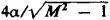. This formula is also valid for thin profiles of arbitrary shape with a sharp leading edge.

### REFERENCES

Zhukovskii, N. E. “O prisoedinennykh vikhriakh.” Izbr. soch., vol. 2. Moscow-Leningrad, 1948.
Loitsianskii, L. G. Mekhanika zhidkosti i gaza, 2nd ed. Moscow, 1957.
Golubev, V. V. Lektsii po teorii kryla. Moscow-Leningrad, 1949.
Abramovich, G. N. Prikladnaia gazovaia dinamika, 2nd ed. Moscow, 1953.
Ferri, A. Aerodinamika sverkhzvukovykh techenii. Moscow, 1953. (Translated from English.)

M. IA. IUDELOVICH

The Great Soviet Encyclopedia, 3rd Edition (1970-1979). © 2010 The Gale Group, Inc. All rights reserved.

## What does it mean when you dream about being lifted?

Being lifted in a dream may indicate that the dreamer is rising above unpleasant conditions or issues. Being lifted can also have the same connotations as ascension and height.

## lift

[lift]
(fluid mechanics)
(mechanical engineering)
(mining engineering)
The vertical height traveled by a cage in a shaft.
The distance between the first level and the surface or between any two levels.
Any of the various gangways from which coal is raised at a slope colliery.
McGraw-Hill Dictionary of Scientific & Technical Terms, 6E, Copyright © 2003 by The McGraw-Hill Companies, Inc.

## lift

1. An elevator used on the stage of a theater, in the orchestra pit, or on the apron.
2. British term for elevator.
3. A handle or projection from the lower sash in a hung window, used as a grip in raising the sash; also called a sash lift.
4. One of a number of frames of scaffolding erected one above another in a vertical direction.
5. The concrete placed between two consecutive horizontal construction joints; usually consists of several layers or courses.
6. In reinforced concrete construction, that portion of a wall, pier, abutment, etc., placed in a single pour.
7. The amount of grouting or mortar placed at a single time in a building structure.
8. In a multi-level excavation, a bench or step.
McGraw-Hill Dictionary of Architecture and Construction. Copyright © 2003 by McGraw-Hill Companies, Inc.

## lifti. That component of the aerodynamic forces acting on the wing or airfoil section that opposes gravity. It is perpendicular to the flight path or free-stream flow. Simply put, it is the difference between the static pressure on the airfoil lower surface and that on the airfoil top surface. Lift (L) = coefficient of lift (CL) ½ × density (ρ) × velocity square (V2) × surface area of airfoil (S), or L = CL ½ρV2S.
ii. The whole or part of an airborne operation, as in the Berlin Airlift. The terms first lift and second lift also are used in the same sense.